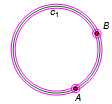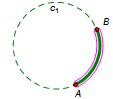# Arc on CircleSelection prerequisites: Either of the following:

One circle and two points on the circle

One center point and two other points equally distant from the center point

This Construct menu command constructs an arc of the given circle bounded by the two points, or an arc centered on the first selected point and bounded by the other two points.

The arc is constructed counter-clockwise from the first selected bounding point to the second. The order in which you select the bounding points determines whether the constructed arc is a minor or major arc.

 Selected Objects: Resulting Construction:One circle, two points on circleArcThree points, with D and E equally distant from CArc